# N B This lecture uses a leftrecursive version

• Slides: 35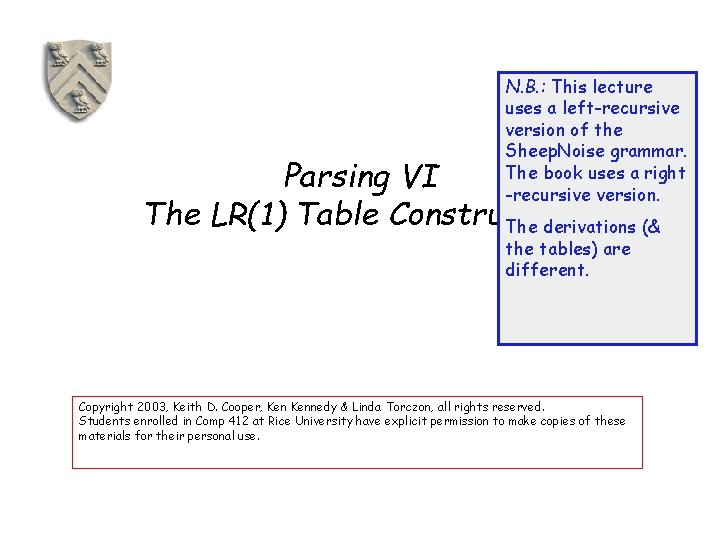N. B. : This lecture uses a left-recursive version of the Sheep. Noise grammar. The book uses a right -recursive version. Parsing VI The LR(1) Table Construction The derivations (& the tables) are different. Copyright 2003, Keith D. Cooper, Kennedy & Linda Torczon, all rights reserved. Students enrolled in Comp 412 at Rice University have explicit permission to make copies of these materials for their personal use.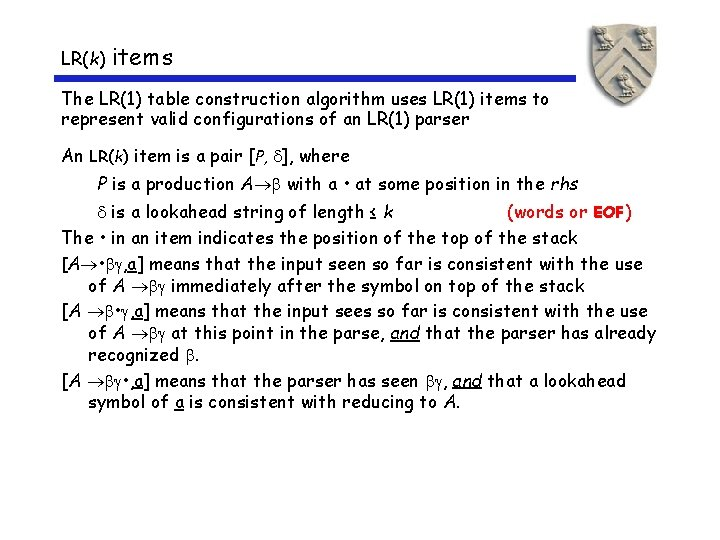LR(k) items The LR(1) table construction algorithm uses LR(1) items to represent valid configurations of an LR(1) parser An LR(k) item is a pair [P, ], where P is a production A with a • at some position in the rhs is a lookahead string of length ≤ k (words or EOF) The • in an item indicates the position of the top of the stack [A • , a] means that the input seen so far is consistent with the use of A immediately after the symbol on top of the stack [A • , a] means that the input sees so far is consistent with the use of A at this point in the parse, and that the parser has already recognized . [A • , a] means that the parser has seen , and that a lookahead symbol of a is consistent with reducing to A.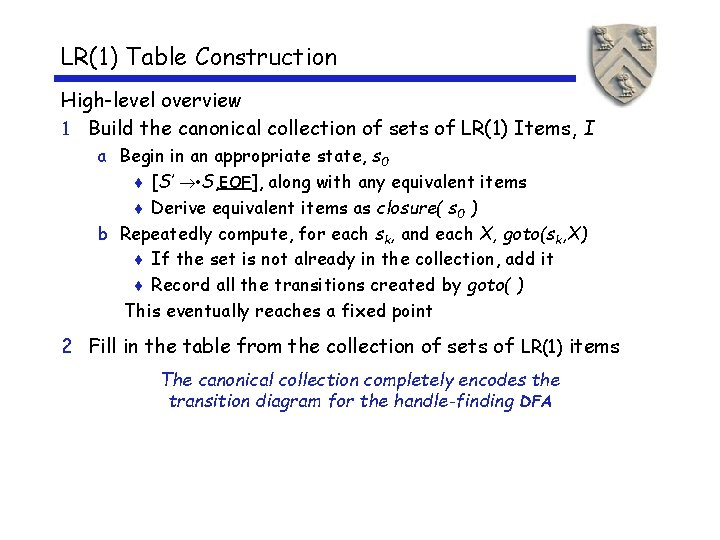LR(1) Table Construction High-level overview 1 Build the canonical collection of sets of LR(1) Items, I a Begin in an appropriate state, s 0 ¨ [S’ • S, EOF], along with any equivalent items ¨ Derive equivalent items as closure( s 0 ) b Repeatedly compute, for each sk, and each X, goto(sk, X) ¨ If the set is not already in the collection, add it ¨ Record all the transitions created by goto( ) This eventually reaches a fixed point 2 Fill in the table from the collection of sets of LR(1) items The canonical collection completely encodes the transition diagram for the handle-finding DFA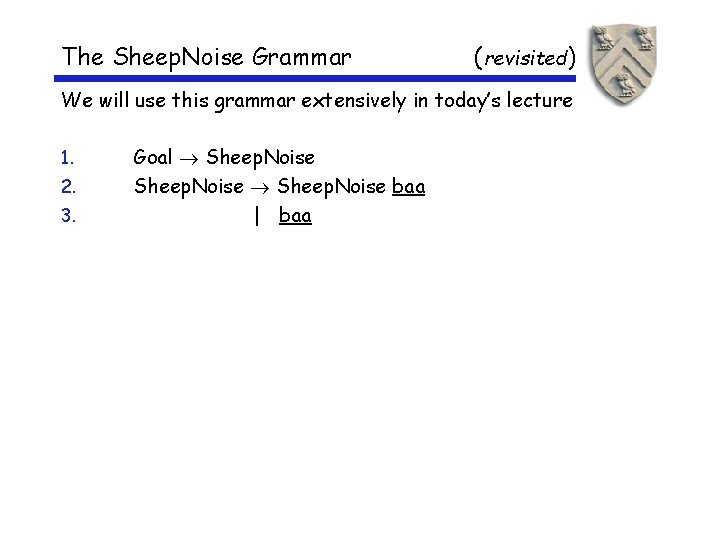The Sheep. Noise Grammar (revisited) We will use this grammar extensively in today’s lecture 1. 2. 3. Goal Sheep. Noise baa | baa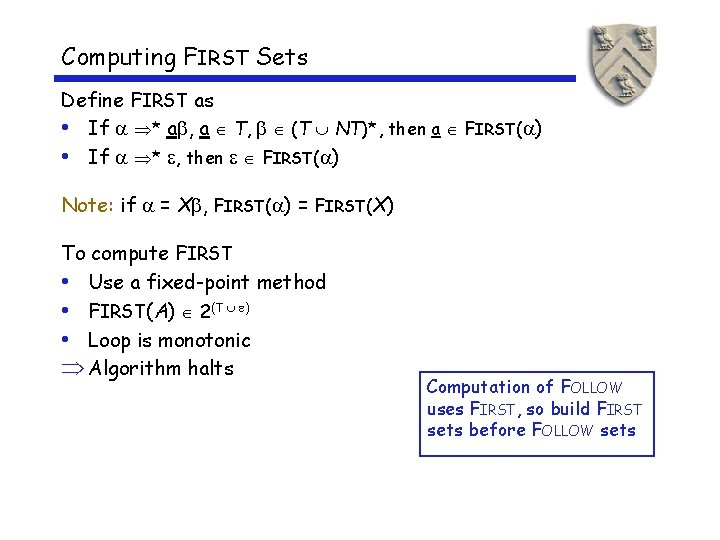Computing FIRST Sets Define FIRST as • If * a , a T, (T NT)*, then a FIRST( ) • If * , then FIRST( ) Note: if = X , FIRST( ) = FIRST(X) To compute FIRST • Use a fixed-point method • FIRST(A) 2(T ) • Loop is monotonic Algorithm halts Computation of FOLLOW uses FIRST, so build FIRST sets before FOLLOW setsComputing FIRST Sets for each x T, FIRST(x) { x } for each A NT, FIRST(A) Ø while (FIRST sets are still changing) for each p P, of the form A , if is then FIRST(A) { } on. SN G on SN baa else if is B 1 B 2…Bk then begin FIRST(A) ( FIRST(B 1) – { } ) for i 1 to k– 1 by 1 while FIRST(Bi ) FIRST(A) ( FIRST(Bi +1) – { } ) if i = k– 1 and FIRST(Bk ) then FIRST(A) { } end For Sheep. Noise: FIRST(Goal) = { baa } FIRST(SN ) = { baa } FIRST(baa) = { baa }Computing FOLLOW Sets FOLLOW(S) {EOF } for each A NT, FOLLOW(A) Ø while (FOLLOW sets are still changing) for each p P, of the form A 1 2 … k FOLLOW( k) FOLLOW(A) TRAILER FOLLOW(A) for i k down to 2 if FIRST( i ) then FOLLOW( i-1 ) FOLLOW( i-1) { FIRST( i ) – { } } TRAILER else FOLLOW( i-1 ) FOLLOW( i-1) FIRST( i ) TRAILER Ø For Sheep. Noise: FOLLOW(Goal ) = { EOF } FOLLOW(SN) = { baa, EOF }Computing Closures Closure(s) adds all the items implied by items already in s • Any item [A B , a] implies [B , x] for each production with B on the lhs, and each x FIRST( a) • Since B is valid, any way to derive B is valid, too The algorithm Closure( s ) while ( s is still changing ) items [A • B , a] s productions B P b FIRST( a) // might be if [B • , b] s then add [B • , b] to s Ø Classic fixed-point method Ø Halts because s ITEMS Ø Worklist version is faster Closure “fills out” a state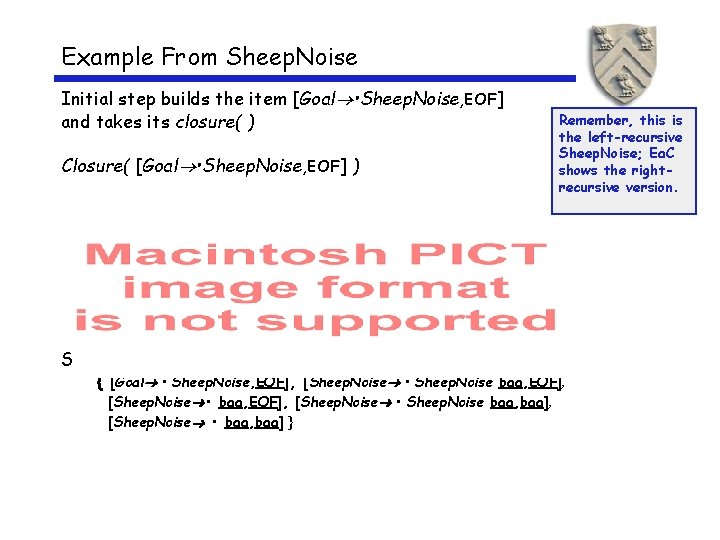Example From Sheep. Noise Initial step builds the item [Goal • Sheep. Noise, EOF] and takes its closure( ) Closure( [Goal • Sheep. Noise, EOF] ) So, S 0 is Remember, this is the left-recursive Sheep. Noise; Ea. C shows the rightrecursive version. { [Goal • Sheep. Noise, EOF], [Sheep. Noise • Sheep. Noise baa, EOF], [Sheep. Noise • baa, EOF], [Sheep. Noise • Sheep. Noise baa, baa], [Sheep. Noise • baa, baa] }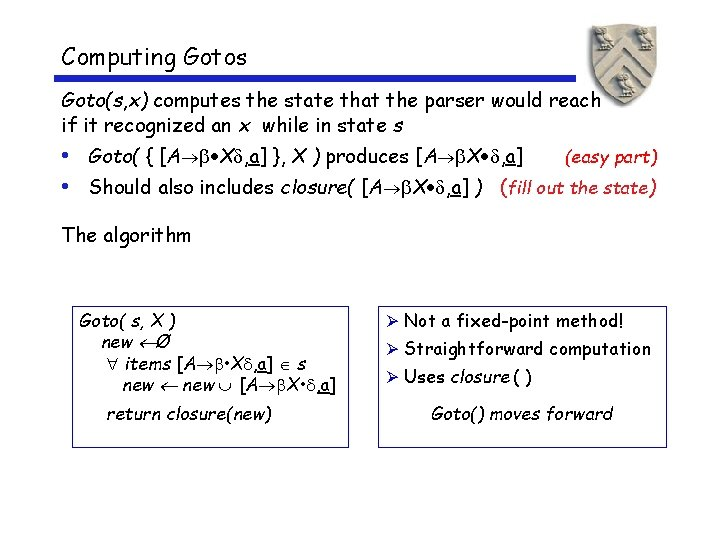Computing Gotos Goto(s, x) computes the state that the parser would reach if it recognized an x while in state s • Goto( { [A X , a] }, X ) produces [A X , a] (easy part) • Should also includes closure( [A X , a] ) (fill out the state) The algorithm Goto( s, X ) new Ø items [A • X , a] s new [A X • , a] return closure(new) Ø Not a fixed-point method! Ø Straightforward computation Ø Uses closure ( ) Goto() moves forward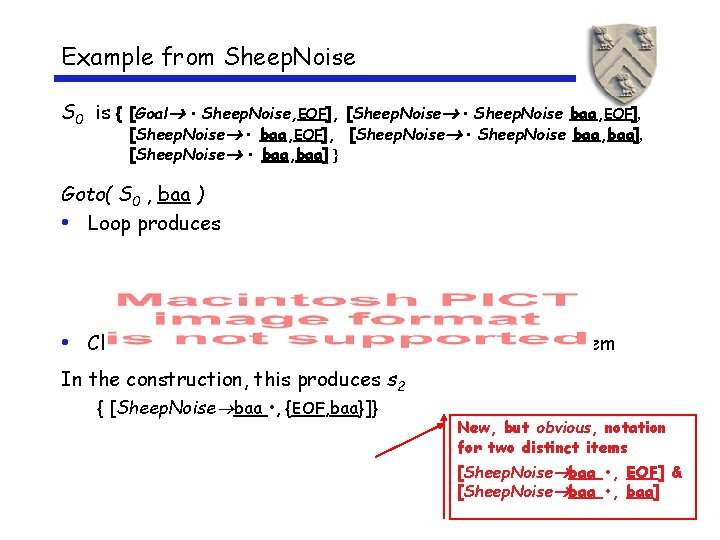Example from Sheep. Noise S 0 is { [Goal • Sheep. Noise, EOF], [Sheep. Noise • Sheep. Noise baa, baa], [Sheep. Noise • baa, baa] } Goto( S 0 , baa ) • Loop produces • Closure adds nothing since • is at end of rhs in each item In the construction, this produces s 2 { [Sheep. Noise baa • , {EOF, baa}]} New, but obvious, notation for two distinct items [Sheep. Noise baa • , EOF] & [Sheep. Noise baa • , baa]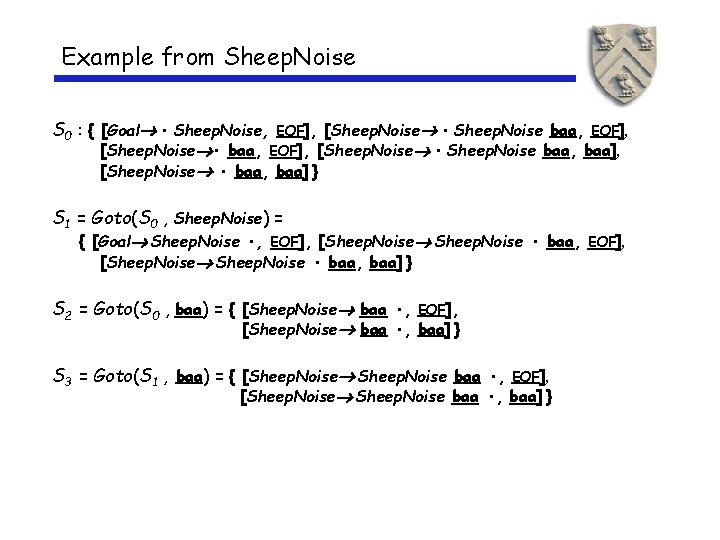Example from Sheep. Noise S 0 : { [Goal • Sheep. Noise, EOF], [Sheep. Noise • Sheep. Noise baa, EOF], [Sheep. Noise • baa, EOF], [Sheep. Noise • Sheep. Noise baa, baa], [Sheep. Noise • baa, baa] } S 1 = Goto(S 0 , Sheep. Noise) = { [Goal Sheep. Noise • , EOF], [Sheep. Noise • baa, baa] } S 2 = Goto(S 0 , baa) = { [Sheep. Noise baa • , EOF], [Sheep. Noise baa • , baa] } S 3 = Goto(S 1 , baa) = { [Sheep. Noise baa • , EOF], [Sheep. Noise baa • , baa] }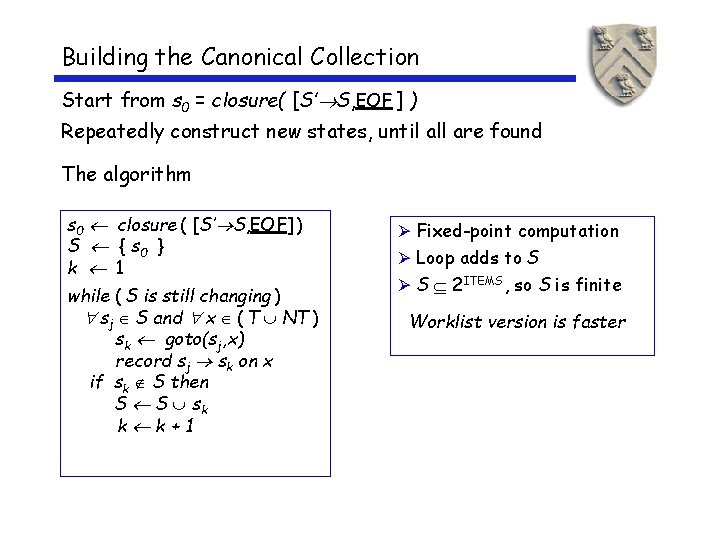Building the Canonical Collection Start from s 0 = closure( [S’ S, EOF ] ) Repeatedly construct new states, until all are found The algorithm s 0 closure ( [S’ S, EOF] ) S { s 0 } k 1 while ( S is still changing ) sj S and x ( T NT ) sk goto(sj, x) record sj sk on x if sk S then S S sk k k+1 Ø Fixed-point computation Ø Loop adds to S Ø S 2 ITEMS, so S is finite Worklist version is fasterExample from Sheep. Noise Starts with S 0 : { [Goal • Sheep. Noise, EOF], [Sheep. Noise • Sheep. Noise baa, EOF], [Sheep. Noise • baa, EOF], [Sheep. Noise • Sheep. Noise baa, baa], [Sheep. Noise • baa, baa] }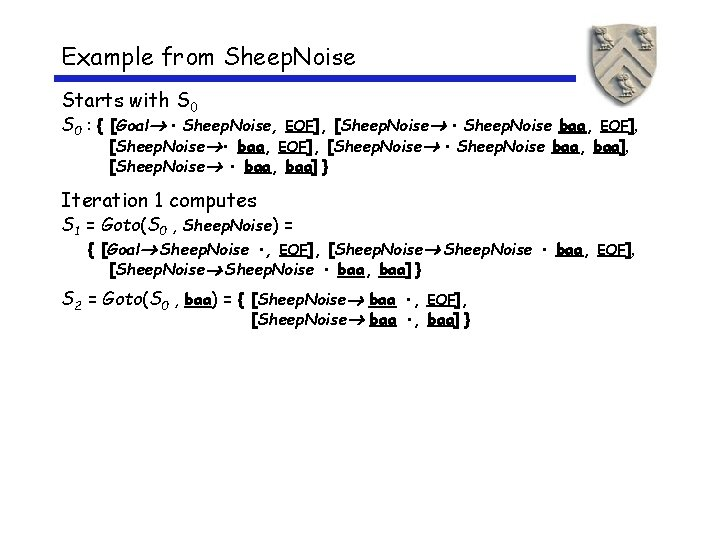Example from Sheep. Noise Starts with S 0 : { [Goal • Sheep. Noise, EOF], [Sheep. Noise • Sheep. Noise baa, EOF], [Sheep. Noise • baa, EOF], [Sheep. Noise • Sheep. Noise baa, baa], [Sheep. Noise • baa, baa] } Iteration 1 computes S 1 = Goto(S 0 , Sheep. Noise) = { [Goal Sheep. Noise • , EOF], [Sheep. Noise • baa, baa] } S 2 = Goto(S 0 , baa) = { [Sheep. Noise baa • , EOF], [Sheep. Noise baa • , baa] }Example from Sheep. Noise Starts with S 0 : { [Goal • Sheep. Noise, EOF], [Sheep. Noise • Sheep. Noise baa, EOF], [Sheep. Noise • baa, EOF], [Sheep. Noise • Sheep. Noise baa, baa], [Sheep. Noise • baa, baa] } Iteration 1 computes S 1 = Goto(S 0 , Sheep. Noise) = { [Goal Sheep. Noise • , EOF], [Sheep. Noise • baa, baa] } S 2 = Goto(S 0 , baa) = { [Sheep. Noise baa • , EOF], [Sheep. Noise baa • , baa] } Iteration 2 computes S 3 = Goto(S 1 , baa) = { [Sheep. Noise baa • , EOF], [Sheep. Noise baa • , baa] }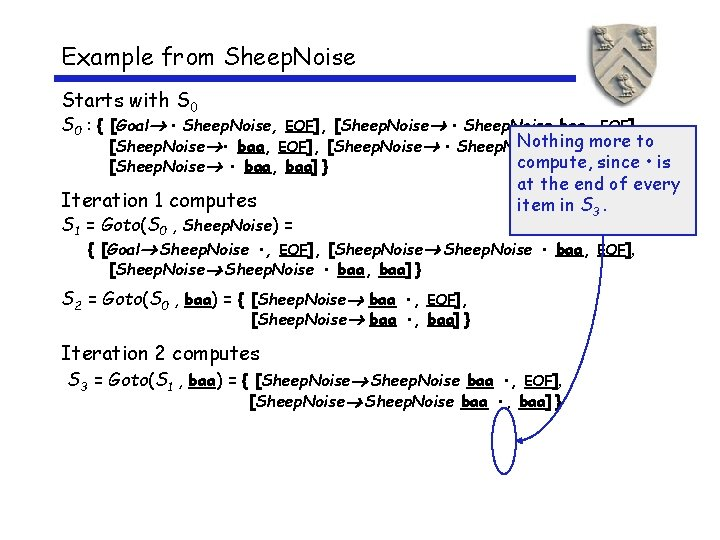Example from Sheep. Noise Starts with S 0 : { [Goal • Sheep. Noise, EOF], [Sheep. Noise • Sheep. Noise baa, EOF], Nothing [Sheep. Noise • baa, EOF], [Sheep. Noise • Sheep. Noise baa, more baa], to compute, since • is at the end of every item in S 3. [Sheep. Noise • baa, baa] } Iteration 1 computes S 1 = Goto(S 0 , Sheep. Noise) = { [Goal Sheep. Noise • , EOF], [Sheep. Noise • baa, baa] } S 2 = Goto(S 0 , baa) = { [Sheep. Noise baa • , EOF], [Sheep. Noise baa • , baa] } Iteration 2 computes S 3 = Goto(S 1 , baa) = { [Sheep. Noise baa • , EOF], [Sheep. Noise baa • , baa] }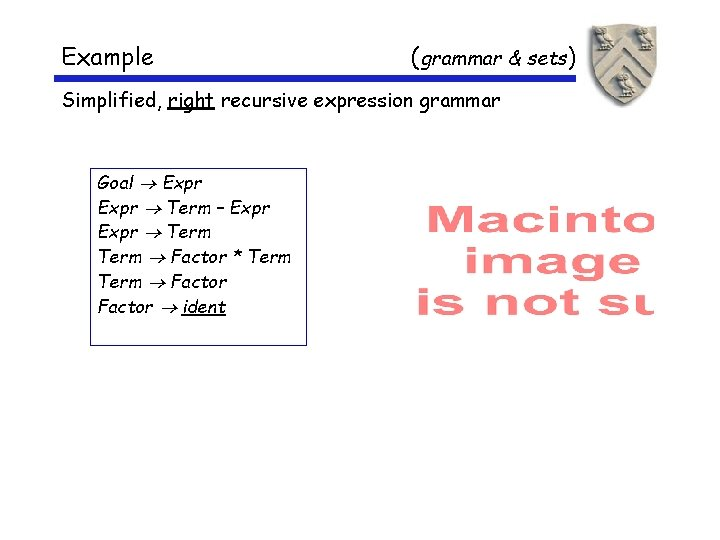Example ( grammar & sets) Simplified, right recursive expression grammar Goal Expr Term – Expr Term Factor * Term Factor ident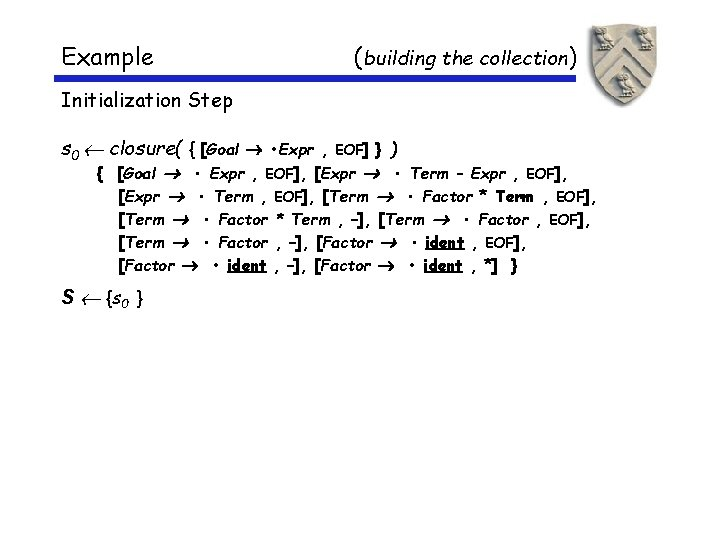Example (building the collection) Initialization Step s 0 closure( { [Goal • Expr , EOF] } ) { [Goal • Expr , EOF], [Expr • Term – Expr , EOF], [Expr • Term , EOF], [Term • Factor * Term , –], [Term • Factor , EOF], [Term • Factor , –], [Factor • ident , EOF], [Factor • ident , –], [Factor • ident , *] } S {s 0 }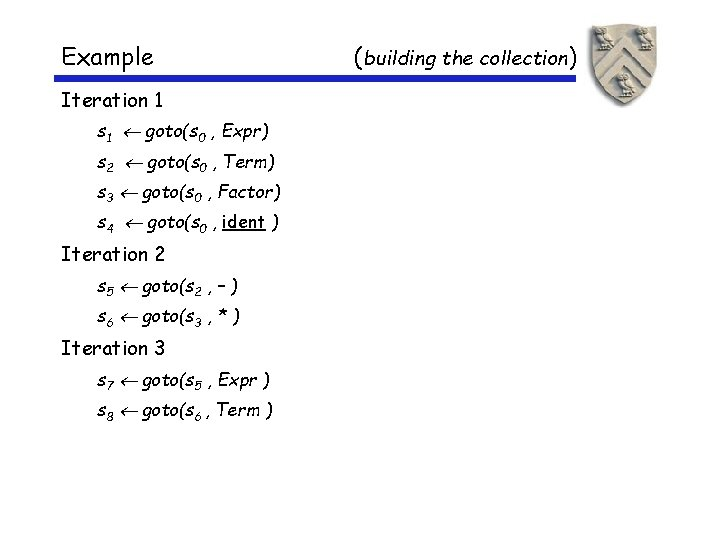Example Iteration 1 s 1 goto(s 0 , Expr) s 2 goto(s 0 , Term) s 3 goto(s 0 , Factor) s 4 goto(s 0 , ident ) Iteration 2 s 5 goto(s 2 , – ) s 6 goto(s 3 , * ) Iteration 3 s 7 goto(s 5 , Expr ) s 8 goto(s 6 , Term ) (building the collection)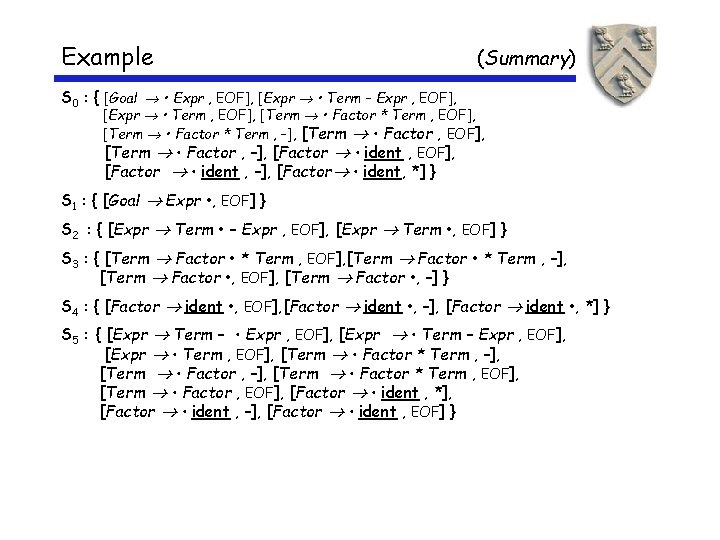Example (Summary) S 0 : { [Goal • Expr , EOF], [Expr • Term – Expr , EOF], [Expr • Term , EOF], [Term • Factor * Term , –], [Term • Factor , EOF], [Term • Factor , –], [Factor • ident , EOF], [Factor • ident , –], [Factor • ident, *] } S 1 : { [Goal Expr • , EOF] } S 2 : { [Expr Term • – Expr , EOF], [Expr Term • , EOF] } S 3 : { [Term Factor • * Term , EOF], [Term Factor • * Term , –], [Term Factor • , EOF], [Term Factor • , –] } S 4 : { [Factor ident • , EOF], [Factor ident • , –], [Factor ident • , *] } S 5 : { [Expr Term – • Expr , EOF], [Expr • Term – Expr , EOF], [Expr • Term , EOF], [Term • Factor * Term , –], [Term • Factor * Term , EOF], [Term • Factor , EOF], [Factor • ident , *], [Factor • ident , –], [Factor • ident , EOF] }Example ( Summary) S 6 : { [Term Factor * • Term , EOF], [Term Factor * • Term , –], [Term • Factor * Term , EOF], [Term • Factor * Term , –], [Term • Factor , EOF], [Term • Factor , –], [Factor • ident , EOF], [Factor • ident , –], [Factor • ident , *] } S 7: { [Expr Term – Expr • , EOF] } S 8 : { [Term Factor * Term • , EOF], [Term Factor * Term • , –] }Example (Summary) The Goto Relationship (from the construction)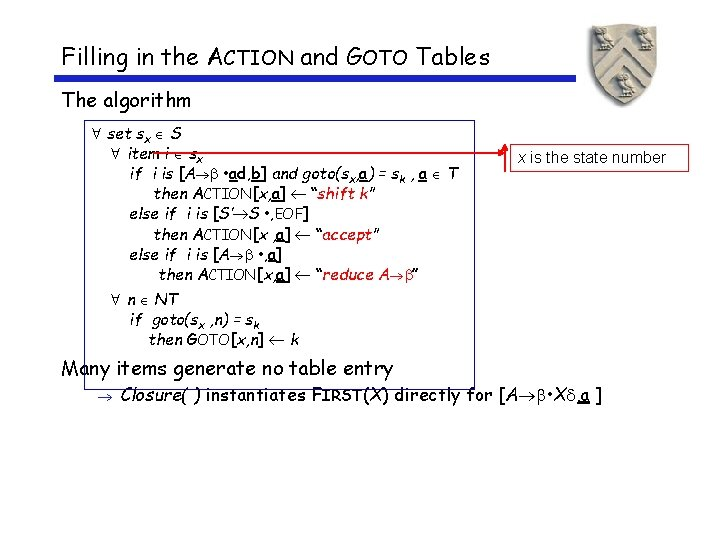Filling in the ACTION and GOTO Tables The algorithm set sx S item i sx if i is [A • ad, b] and goto(sx, a) = sk , a T then ACTION[x, a] “shift k” else if i is [S’ S • , EOF] then ACTION[x , a] “accept” else if i is [A • , a] then ACTION[x, a] “reduce A ” x is the state number n NT if goto(sx , n) = sk then GOTO[x, n] k Many items generate no table entry Closure( ) instantiates FIRST(X) directly for [A • X , a ]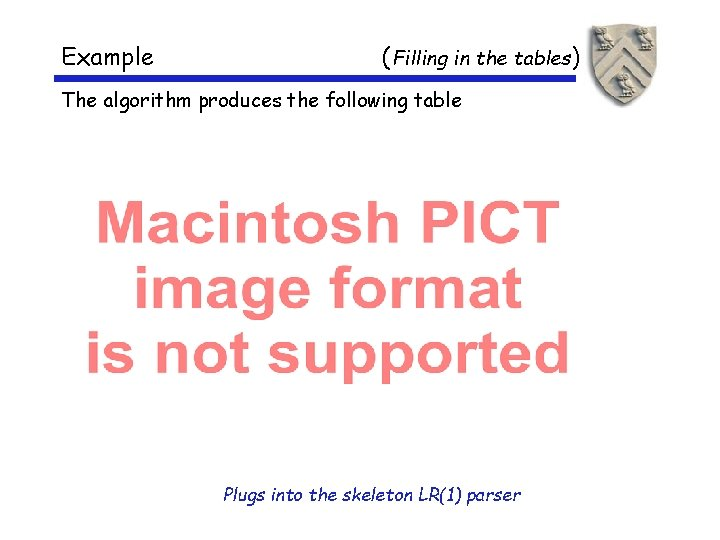Example ( Filling in the tables) The algorithm produces the following table Plugs into the skeleton LR(1) parser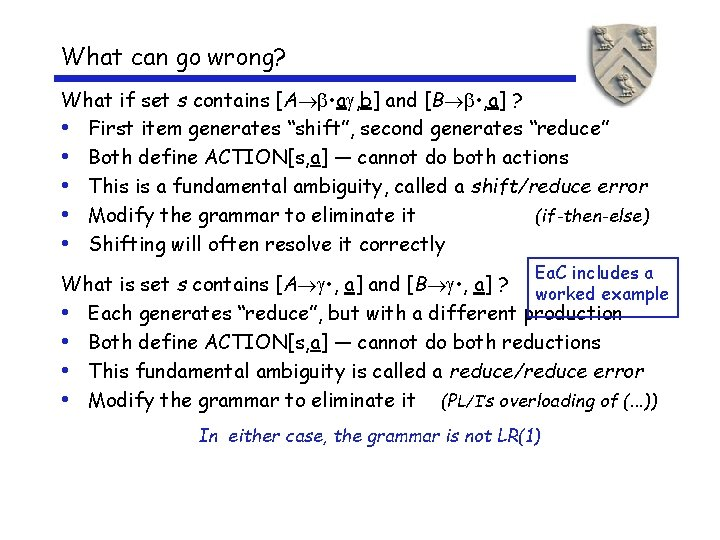What can go wrong? What if set s contains [A • a , b] and [B • , a] ? • First item generates “shift”, second generates “reduce” • Both define ACTION[s, a] — cannot do both actions • This is a fundamental ambiguity, called a shift/reduce error • Modify the grammar to eliminate it (if-then-else) • Shifting will often resolve it correctly Ea. C includes a What is set s contains [A • , a] and [B • , a] ? worked example • Each generates “reduce”, but with a different production • Both define ACTION[s, a] — cannot do both reductions • This fundamental ambiguity is called a reduce/reduce error • Modify the grammar to eliminate it (PL/I’s overloading of (. . . )) In either case, the grammar is not LR(1)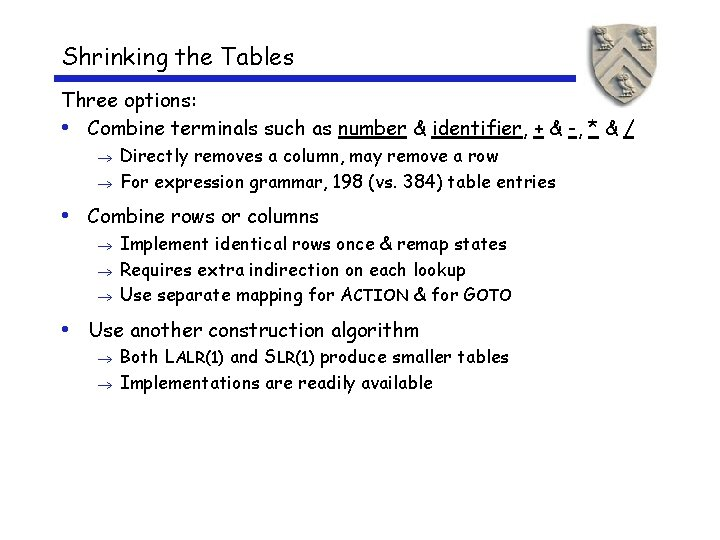Shrinking the Tables Three options: • Combine terminals such as number & identifier, + & -, * & / Directly removes a column, may remove a row For expression grammar, 198 (vs. 384) table entries • Combine rows or columns Implement identical rows once & remap states Requires extra indirection on each lookup Use separate mapping for ACTION & for GOTO • Use another construction algorithm Both LALR(1) and SLR(1) produce smaller tables Implementations are readily availableLR(k) versus LL(k) (Top-down Recursive Descent ) Finding Reductions LR(k) Each reduction in the parse is detectable with 1 the complete left context, 2 the reducible phrase, itself, and 3 the k terminal symbols to its right LL(k) Parser must select the reduction based on 1 The complete left context 2 The next k terminals Thus, LR(k) examines more context “… in practice, programming languages do not actually seem to fall in the gap between LL(1) languages and deterministic languages” J. J. Horning, “LR Grammars and Analysers”, in Compiler Construction, An Advanced Course, Springer-Verlag, 1976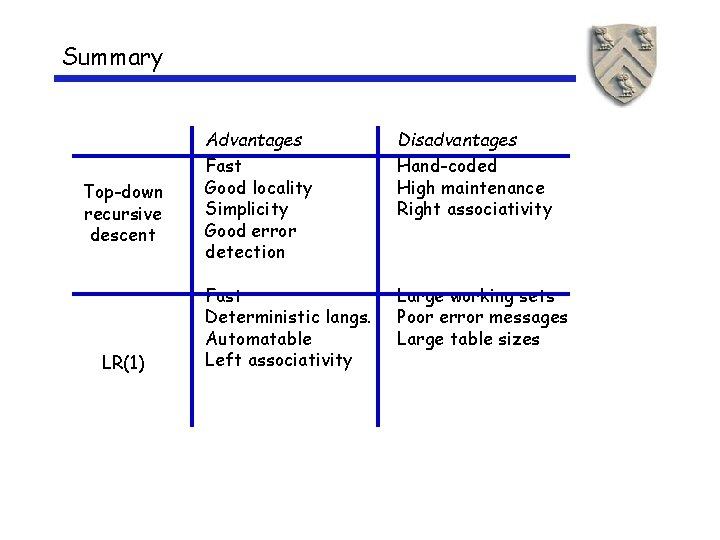Summary Top-down recursive descent LR(1) Advantages Fast Good locality Simplicity Good error detection Disadvantages Hand-coded High maintenance Right associativity Fast Deterministic langs. Automatable Left associativity Large working sets Poor error messages Large table sizesExtra Slides Start Here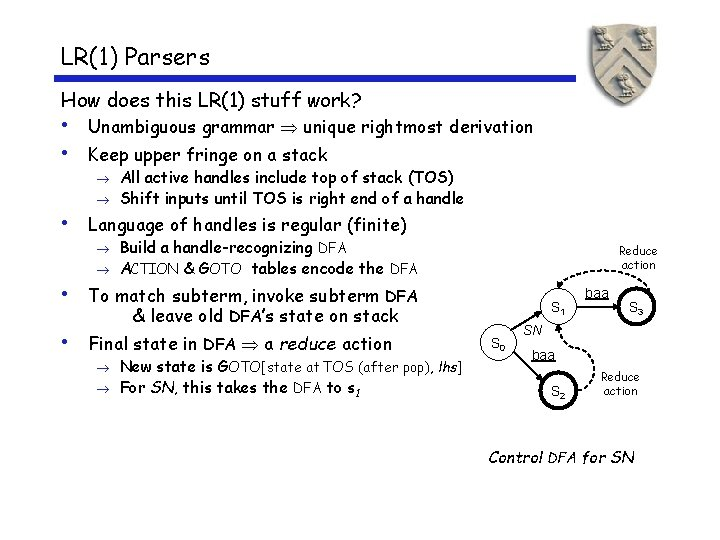LR(1) Parsers How does this LR(1) stuff work? • Unambiguous grammar unique rightmost derivation • Keep upper fringe on a stack All active handles include top of stack (TOS) Shift inputs until TOS is right end of a handle • Language of handles is regular (finite) Build a handle-recognizing DFA ACTION & GOTO tables encode the DFA Reduce action • To match subterm, invoke subterm DFA S 1 & leave old DFA’s state on stack • Final state in DFA a reduce action New state is GOTO[state at TOS (after pop), lhs] For SN, this takes the DFA to s 1 S 0 baa S 3 SN baa S 2 Reduce action Control DFA for SNBuilding LR(1) Parsers How do we generate the ACTION and GOTO tables? • Use the grammar to build a model of the DFA • Use the model to build ACTION & GOTO tables • If construction succeeds, the grammar is LR(1) The Big Picture • Model the state of the parser • Use two functions goto( s, X ) and closure( s ) Terminal or non-terminal goto() is analogous to move() in the subset construction closure() adds information to round out a state • Build up the states and transition functions of the DFA • Use this information to fill in the ACTION and GOTO tablesLR(1) Items The production A , where = B 1 B 1 B 1 with lookahead a, can give rise to 4 items [A • B 1 B 2 B 3, a], [A B 1 • B 2 B 3, a], [A B 1 B 2 • B 3, a], & [A B 1 B 2 B 3 • , a] The set of LR(1) items for a grammar is finite What’s the point of all these lookahead symbols? • Carry them along to choose the correct reduction, if there is a choice • Lookaheads are bookkeeping, unless item has • at right end Has no direct use in [A • , a] In [A • , a], a lookahead of a implies a reduction by A For { [A • , a], [B • , b] }, a reduce to A; FIRST( ) shift Limited right context is enough to pick the actions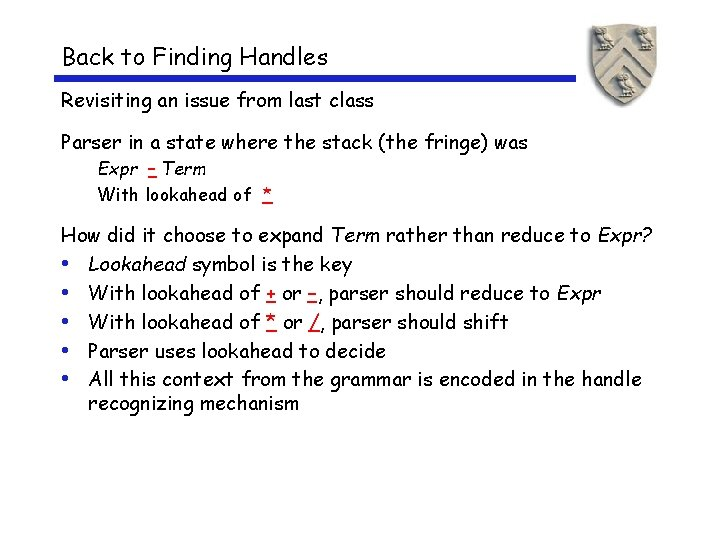Back to Finding Handles Revisiting an issue from last class Parser in a state where the stack (the fringe) was Expr – Term With lookahead of * How did it choose to expand Term rather than reduce to Expr? • Lookahead symbol is the key • With lookahead of + or –, parser should reduce to Expr • With lookahead of * or /, parser should shift • Parser uses lookahead to decide • All this context from the grammar is encoded in the handle recognizing mechanismRemember this slide from last lecture? Back to x – 2 * y shift here reduce here 1. Shift until TOS is the right end of a handle 2. Find the left end of the handle & reduce